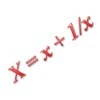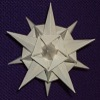# Search by Topic

#### Resources tagged with Polynomial functions and their roots similar to What's Your Mean?:

Filter by: Content type:
Age range:
Challenge level:

### There are 18 results

Broad Topics > Functions and Graphs > Polynomial functions and their roots### Spinners

##### Age 16 to 18 Challenge Level:

How do scores on dice and factors of polynomials relate to each other?### Mechanical Integration

##### Age 16 to 18 Challenge Level:

To find the integral of a polynomial, evaluate it at some special points and add multiples of these values.### Fibonacci Fashion

##### Age 16 to 18 Challenge Level:

What have Fibonacci numbers to do with solutions of the quadratic equation x^2 - x - 1 = 0 ?### An Introduction to Galois Theory

##### Age 16 to 18

This article only skims the surface of Galois theory and should probably be accessible to a 17 or 18 year old school student with a strong interest in mathematics.### The Why and How of Substitution

##### Age 16 to 18

Step back and reflect! This article reviews techniques such as substitution and change of coordinates which enable us to exploit underlying structures to crack problems.### Agile Algebra

##### Age 16 to 18 Challenge Level:

Observe symmetries and engage the power of substitution to solve complicated equations.### Polynomial Interpolation

##### Age 16 to 18 Challenge Level:

Can you fit polynomials through these points?### Interpolating Polynomials

##### Age 16 to 18 Challenge Level:

Given a set of points (x,y) with distinct x values, find a polynomial that goes through all of them, then prove some results about the existence and uniqueness of these polynomials.### Weekly Challenge 39: Symmetrically So

##### Age 16 to 18 Challenge Level:

Exploit the symmetry and turn this quartic into a quadratic.### Root to Poly

##### Age 14 to 16 Challenge Level:

Find the polynomial p(x) with integer coefficients such that one solution of the equation p(x)=0 is $1+\sqrt 2+\sqrt 3$.### More Polynomial Equations

##### Age 16 to 18 Challenge Level:

Find relationships between the polynomials a, b and c which are polynomials in n giving the sums of the first n natural numbers, squares and cubes respectively.### Polynomial Relations

##### Age 16 to 18 Challenge Level:

Given any two polynomials in a single variable it is always possible to eliminate the variable and obtain a formula showing the relationship between the two polynomials. Try this one.### Two Cubes

##### Age 14 to 16 Challenge Level:

Two cubes, each with integral side lengths, have a combined volume equal to the total of the lengths of their edges. How big are the cubes? [If you find a result by 'trial and error' you'll need to. . . .### Common Divisor

##### Age 14 to 16 Challenge Level:

Find the largest integer which divides every member of the following sequence: 1^5-1, 2^5-2, 3^5-3, ... n^5-n.### Janine's Conjecture

##### Age 14 to 16 Challenge Level:

Janine noticed, while studying some cube numbers, that if you take three consecutive whole numbers and multiply them together and then add the middle number of the three, you get the middle number. . . .### Poly Fibs

##### Age 16 to 18 Challenge Level:

A sequence of polynomials starts 0, 1 and each poly is given by combining the two polys in the sequence just before it. Investigate and prove results about the roots of the polys.### Telescoping Functions

##### Age 16 to 18

Take a complicated fraction with the product of five quartics top and bottom and reduce this to a whole number. This is a numerical example involving some clever algebra.